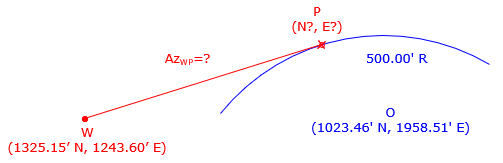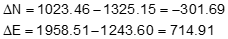### D. Examples

#### 1. Three point curve

Three known coordinate points are

 Point North (ft) East (ft) A 902.72 1751.91 B 870.76 1237.76 C 538.57 1293.16

Determine the radius of the circle passing through the points and coordinates of the radius point.

SketchCompute the coefficients using Equations I-3 through I-6Use the coefficients in Equations I-1 and I-2 to get radius point coordinatesUsing point ACheck using point BChecks within rounding.

#### 2. Tangent line

What is the direction of the line line tangent to the arc shown below? What are the coordinates of the intersection point?Inverse from point W to the radius point OUsing Equation I-12 compute the angle g between the line to the radius point and tangent line.Determine the azimuth and length of the tangent line

.Since the tangent line is left of the line to the radius point, to get its azimuth subtract g from AzWO' Equation, I-16.Its length comes from Equation I-13Forward Computation, Equations I-17 and I-18, to compute tangent point coordinatesTangent azimuth 72°45'42" Tangent pt coords 1501.00' N 1810.34' E

#### 3. Three-tangent arc

What is the radius of the arc that is tangent to the three line shown?Label the geometryEstablsh points G and H using direction-direction intersections (results shown, comps left to user)

 Point North East G 1849.672 1305.139 H 1735.850 1670.923

ΔG and ΔH from the azimuths (comps left to user)

 ΔG 63°05'03" ΔH 49°03'40"

Inverse Computation to obtain distance from point G to point H  (comps left to user): 383.084

Apply Equation I-19 to get the arc radiusTangent distance for first arc section (comps left to user): 219.721

Radius point coordinates (comps left to user): 1,442.592' N and 1,408.580' E